# Probabilistic logics in machine learning

### Fabrizio Riguzzi

##### http://cplint.lamping.unife.it# Outline

• Logic

• Probabilistic logics

• Probabilistic logic programming

• Applications

• Inference

• Learning

• Examples

# Logic

• Useful to model domains with complex relationships among entities

• Various forms:

• First Order Logic

• Logic Programming

• Description Logics

# First Order Logic

• Very expressive

• Open World Assumption

• Undecidable

$\begin{array}{l} \forall x\ Intelligent(x)\rightarrow GoodMarks(x)\\ \forall x,y\ Friends(x,y)\rightarrow (Intelligent(x)\leftrightarrow Intelligent(y)) \end{array}$

# Logic Programming

• A subset of First Order Logic

• Closed World Assumption

• Turing complete

• Prolog

$\begin{array}{l} \mathit{flu}(bob).\\ hay\_\mathit{fever}(bob).\\ sneezing(X)\leftarrow \mathit{flu}(X).\\ sneezing(X)\leftarrow hay\_\mathit{fever}(X).\\ \end{array}$

# Description Logics

• Subsets of First Order Logic

• Open World Assumption

• Decidable, efficient inference

• Special syntax using concepts (unary predicates) and roles (binary predicates)

\begin{aligned} &&{\mathit{fluffy}}: Cat\\ &&tom : Cat \\ &&Cat \sqsubseteq Pet \\ &&\exists hasAnimal.Pet \sqsubseteq NatureLover\\ &&(kevin, {\mathit{fluffy}}):hasAnimal \\ &&(kevin, tom):hasAnimal \end{aligned}

# Combining Logic and Probability

• Logic does not handle well uncertainty

• Graphical models do not handle well relationships among entities

• Solution: combine the two

• Many approaches proposed in the areas of Logic Programming, Uncertainty in AI, Machine Learning, Databases, Knowledge Representation

# Probabilistic Logic Programming

• Distribution Semantics [Sato ICLP95]

• A probabilistic logic program defines a probability distribution over normal logic programs (called instances or possible worlds or simply worlds)

• The distribution is extended to a joint distribution over worlds and interpretations (or queries)

• The probability of a query is obtained from this distribution

# Probabilistic Logic Programming (PLP) Languages under the Distribution Semantics

• Probabilistic Logic Programs [Dantsin RCLP91]

• Probabilistic Horn Abduction [Poole NGC93], Independent Choice Logic (ICL) [Poole AI97]

• PRISM [Sato ICLP95]

• Logic Programs with Annotated Disjunctions (LPADs) [Vennekens et al. ICLP04]

• ProbLog [De Raedt et al. IJCAI07]

• They differ in the way they define the distribution over logic programs

# Logic Programs with Annotated Disjunctions

$\begin{array}{l} sneezing(X):0.7\vee null:0.3\leftarrow \mathit{flu}(X).\\ sneezing(X):0.8\vee null:0.2\leftarrow hay\_\mathit{fever}(X).\\ \mathit{flu}(bob).\\ hay\_\mathit{fever}(bob).\\ \end{array}$

• Distributions over the head of rules

• $$null$$ does not appear in the body of any rule

• Worlds obtained by selecting one atom from the head of every grounding of each clause

$\begin{array}{ll} sneezing(bob)\leftarrow \mathit{flu}(bob).& null\leftarrow \mathit{flu}(bob).\\ sneezing(bob)\leftarrow hay\_\mathit{fever}(bob).& sneezing(bob)\leftarrow hay\_\mathit{fever}(bob).\\ \mathit{flu}(bob).&\mathit{flu}(bob).\\ hay\_\mathit{fever}(bob).&hay\_\mathit{fever}(bob).\\ P(w_1)=0.7\times 0.8 &P(w_2)=0.3\times 0.8\\ \\ sneezing(bob)\leftarrow \mathit{flu}(bob).& null\leftarrow \mathit{flu}(bob).\\ null\leftarrow hay\_\mathit{fever}(bob).& null\leftarrow hay\_\mathit{fever}(bob).\\ \mathit{flu}(bob).&\mathit{flu}(bob).\\ hay\_\mathit{fever}(bob). &hay\_\mathit{fever}(bob).\\ P(w_3)=0.7\times 0.2 &P(w_4)=0.3\times 0.2 \end{array}$ $\label{ds} P(Q)=\sum_{w\in W_\mathcal{T}}P(Q,w)=\sum_{w \in W_\mathcal{T}}P(Q|w)P(w)=\sum_{w\in W_\mathcal{T}: w\models Q}P(w)$

• $$sneezing(bob)$$ is true in 3 worlds

• $$P(sneezing(bob))=0.7\times 0.8+0.3\times 0.8+0.7\times 0.2=0.94$$

# ProbLog

$\begin{array}{l} sneezing(X)\leftarrow \mathit{flu}(X),\mathit{flu}\_sneezing(X).\\ sneezing(X)\leftarrow hay\_\mathit{fever}(X),hay\_\mathit{fever}\_sneezing(X).\\ \mathit{flu}(bob).\\ hay\_\mathit{fever}(bob).\\ 0.7::\mathit{flu}\_sneezing(X).\\ 0.8::hay\_\mathit{fever}\_sneezing(X).\\ \end{array}$

• Distributions over facts

• Worlds obtained by selecting or not every grounding of each probabilistic fact

# Example Program (ProbLog) Worlds

• 4 worlds

$\begin{array}{l} sneezing(X)\leftarrow \mathit{flu}(X),\mathit{flu}\_sneezing(X).\\ sneezing(X)\leftarrow hay\_\mathit{fever}(X),hay\_\mathit{fever}\_sneezing(X).\\ \mathit{flu}(bob).\\ hay\_\mathit{fever}(bob). \end{array}$

$\begin{array}{ll} \mathit{flu}\_sneezing(bob).\\ hay\_\mathit{fever}\_sneezing(bob).&hay\_\mathit{fever}\_sneezing(bob).\\ P(w_1)=0.7\times 0.8 &P(w_2)=0.3\times 0.8\\ \mathit{flu}\_sneezing(bob).\\ P(w_3)=0.7\times 0.2 &P(w_4)=0.3\times 0.2 \end{array}$

• $$sneezing(bob)$$ is true in 3 worlds

• $$P(sneezing(bob))=0.7\times 0.8+0.3\times 0.8+0.7\times 0.2=0.94$$

# Logic Programs with Annotated Disjunctions

$\begin{array}{l} strong\_sneezing(X):0.3\vee moderate\_sneezing(X):0.4\leftarrow \mathit{flu}(X).\\ strong\_sneezing(X):0.4\vee moderate\_sneezing(X):0.2\leftarrow hay\_\mathit{fever}(X).\\ \mathit{flu}(bob).\\ hay\_\mathit{fever}(bob).\\ \end{array}$

• 9 worlds

• $$P(strong\_sneezing(bob))=?$$

# Expressive Power

• All languages under the distribution semantics have the same expressive power

• LPADs have the most general syntax

• There are transformations that can convert each one into the others

• ProbLog to LPAD: direct mapping

• Clause $$C_i$$ with variables $$\overline{X}$$ $H_1:p_1 \vee\ldots\vee H_n:p_n \leftarrow B.$ is translated into $\begin{array}{l} H_1\leftarrow B,f_{i,1}(\overline{X}).\\ H_2\leftarrow B,not( f_{i,1}(\overline{X})),f_{i,2}(\overline{X}).\\ \vdots\\ H_n\leftarrow B,not(f_{i,1}(\overline{X})),\ldots,not( f_{i,n-1}(\overline{X})).\\ \pi_1::f_{i,1}(\overline{X}).\\ \vdots\\ \pi_{n-1}::f_{i,n-1}(\overline{X}). \end{array}$ where $$\pi_1=p_1$$, $$\pi_2=\frac{p_2}{1-\pi_1}$$, $$\pi_3=\frac{p_3}{(1-\pi_1)(1-\pi_2)},\ldots$$

• In general $$\pi_i=\frac{p_i}{\prod_{j=1}^{i-1}(1-\pi_j)}$$

• Inference: we want to compute the probability of a query given the model and, possibly, some evidence

• Weight learning: we know the structural part of the model (the logic formulas) but not the numeric part (the weights) and we want to infer the weights from data

• Structure learning we want to infer both the structure and the weights of the model from data

# Applications

• Link prediction: given a (social) network, compute the probability of the existence of a link between two entities (UWCSE)advisedby(X, Y) :0.7 :-
publication(P, X),
publication(P, Y),
student(X).

# Applications

• Classify web pages on the basis of the link structure (WebKB)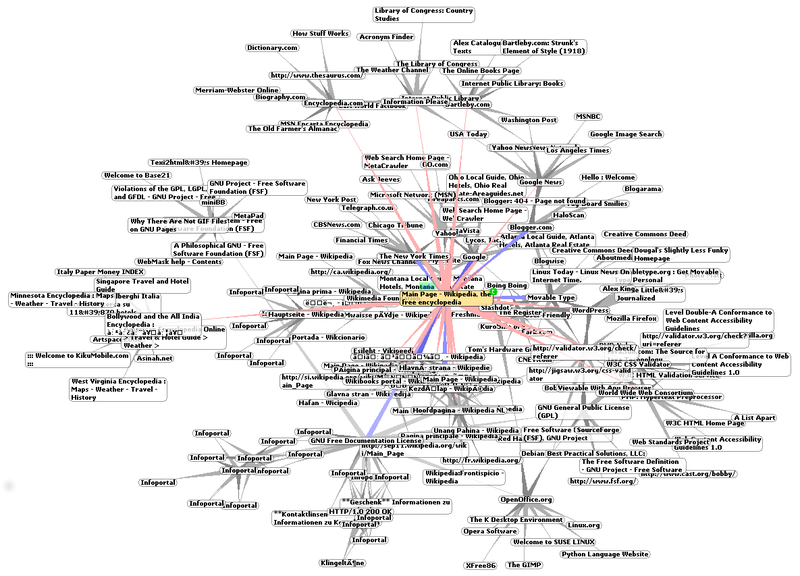coursePage(Page1): 0.3 :- linkTo(Page2,Page1),coursePage(Page2).
...
coursePage(Page): 0.9 :- has('syllabus',Page).
...

# Applications

• Entity resolution: identify identical entities in text or databases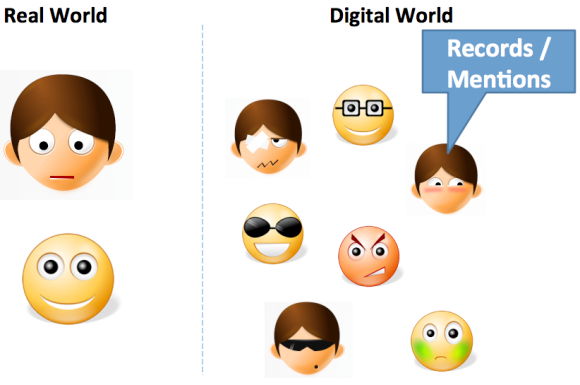samebib(A,B):0.9 :-
samebib(A,C), samebib(C,B).
sameauthor(A,B):0.6 :-
sameauthor(A,C), sameauthor(C,B).
sametitle(A,B):0.7 :-
sametitle(A,C), sametitle(C,B).
samevenue(A,B):0.65 :-
samevenue(A,C), samevenue(C,B).
samebib(B,C):0.5 :-
author(B,D),author(C,E),sameauthor(D,E).
samebib(B,C):0.7 :-
title(B,D),title(C,E),sametitle(D,E).
samebib(B,C):0.6 :-
venue(B,D),venue(C,E),samevenue(D,E).
samevenue(B,C):0.3 :-
haswordvenue(B,logic),
haswordvenue(C,logic).
...

# Applications

• Chemistry: given the chemical composition of a substance, predict its mutagenicity or its carcenogenicity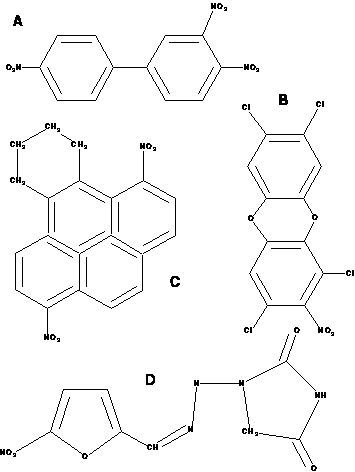active(A):0.4 :-
atm(A,B,c,29,C),
gteq(C,-0.003),
ring_size_5(A,D).
active(A):0.6:-
lumo(A,B), lteq(B,-2.072).
active(A):0.3 :-
bond(A,B,C,2),
bond(A,C,D,1),
ring_size_5(A,E).
active(A):0.7 :-
carbon_6_ring(A,B).
active(A):0.8 :-
anthracene(A,B).
...

# Applications

• Medicine: diagnose diseases on the basis of patient information (Hepatitis), influence of genes on HIV, risk of falling of elderly people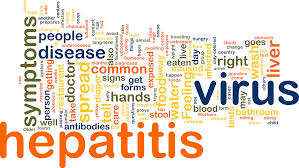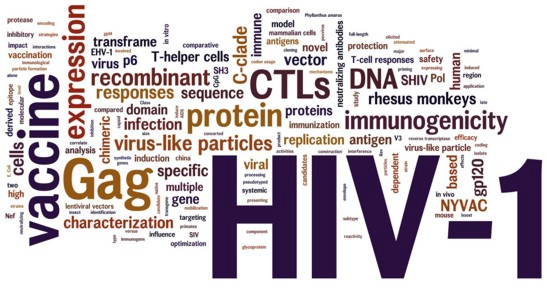# Inference for PLP under DS

• Computing the probability of a query (no evidence)

• Knowledge compilation:

• compile the program to an intermediate representation

• Binary Decision Diagrams (ProbLog [De Raedt et al. IJCAI07], cplint [Riguzzi AIIA07,Riguzzi LJIGPL09], PITA [Riguzzi & Swift ICLP10])

• deterministic, Decomposable Negation Normal Form circuit (d-DNNF) (ProbLog2 [Fierens et al. TPLP15])

• Sentential Decision Diagrams

• compute the probability by weighted model counting

# Inference for PLP under DS

• Bayesian Network based:

• Convert to BN

• Use BN inference algorithms (CVE [Meert et al. ILP09])

• Lifted inference

# Knowledge Compilation

• Assign Boolean random variables to the probabilistic rules

• Given a query $$Q$$, compute its explanations, assignments to the random variables that are sufficient for entailing the query

• Let $$K$$ be the set of all possible explanations

• Build the formula $F(Q)=\bigvee_{\kappa\in K}\bigwedge_{X \in \kappa}X\bigwedge_{\overline{X} \in \kappa}\overline{X}$

• Build a BDD representing $$F(Q)$$

# Binary Decision Diagrams

• A BDD for a function of Boolean variables is a rooted graph that has one level for each Boolean variable

• A node $$n$$ in a BDD has two children: one corresponding to the 1 value of the variable associated with $$n$$ and one corresponding the 0 value of the variable

• The leaves store either 0 or 1.

$F(X_{11},X_{21})=X_{11}\vee X_{21}$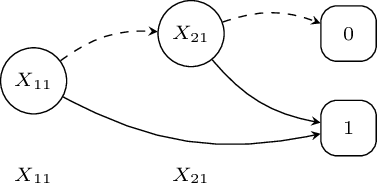# Binary Decision Diagrams$f_K(\mathbf{X})=X_{11}\times f_K^{X_{11}}(\mathbf{X})+\neg X_{11}\times f_K^{\neg X_{11}}(\mathbf{X})$

$P(f_K(\mathbf{X}))=P(X_{11})P(f_K^{X_{11}}(\mathbf{X}))+(1-P(X_{11}))P(f_K^{\neg X_1}(\mathbf{X}))$

# Probability from a BDD

• Dynamic programming algorithm [De Raedt et al 2007]

• Function $$\mathrm{Prob}(n)$$

• if $$n$$ is a terminal note

• return $$value(n)$$

• else

• return $$\mathrm{Prob}(child_1(n)\times p(v(n))+\mathrm{Prob}(child_0(n))\times (1-p(v(n)))$$

# Approximate Inference

• Inference problem is #P hard

• For large models inference is intractable

• Approximate inference

• Monte Carlo: draw samples of the truth value of the query

• Iterative deepening: gives a lower and an upper bound

• Compute only the best $$k$$ explanations: branch and bound, gives a lower bound

# Monte Carlo

• The disjunctive clause

$$C_r=H_1:\alpha_1\vee \ldots\vee H_n:\alpha_n\leftarrow L_1,\ldots,L_m.$$

is transformed into the set of clauses $$MC(C_r)$$

$$\begin{array}{l} MC(C_r,1)=H_1\leftarrow L_1,\ldots,L_m,sample\_head(n,r,VC,NH),NH=1.\\ \ldots\\ MC(C_r,n)=H_n\leftarrow L_1,\ldots,L_m,sample\_head(n,r,VC,NH),NH=n.\\ \end{array}$$

• Sample truth value of query Q:

...
(call(Q)->    NT1 is NT+1 ;   NT1 =NT),
...

# Parameter Learning

• Problem: given a set of interpretations, a program, find the parameters maximizing the likelihood of the interpretations (or of instances of a target predicate)

• The interpretations record the truth value of ground atoms, not of the choice variables

• Unseen data: relative frequency can’t be used

# Parameter Learning

• An Expectation-Maximization algorithm must be used:

• Expectation step: the distribution of the unseen variables in each instance is computed given the observed data

• Maximization step: new parameters are computed from the distributions using relative frequency

• End when likelihood does not improve anymore

# Parameter Learning

•  [Thon et al. ECML 2008] proposed an adaptation of EM for CPT-L, a simplified version of LPADs

•  The algorithm computes the counts efficiently by repeatedly traversing the BDDs representing the explanations

•  [Ishihata et al. ILP 2008] independently proposed a similar algorithm

• LFI-ProbLog [Gutamnn et al. ECML 2011]: EM for ProbLog

• EMBLEM [Riguzzi & Bellodi IDA 2013] adapts [Ishihata et al. ILP 2008] to LPADs

# EMBLEM

• EM over Bdds for probabilistic Logic programs Efficient Mining

• Input: an LPAD; logical interpretations (data); target predicate(s)

• all ground atoms in the interpretations for the target predicate(s) correspond to as many queries

• BDDs encode the explanations for each query $$Q$$

• Expectations computed with two passes over the BDDs

• Given a trivial LPAD or an empty one, a set of interpretations (data)

• Find the model and the parameters that maximize the probability of the data (log-likelihood)

• SLIPCOVER: Structure LearnIng of Probabilistic logic program by searching OVER the clause space EMBLEM [Riguzzi & Bellodi TPLP 2015]

1. Beam search in the space of clauses to find the promising ones

2. Greedy search in the space of probabilistic programs guided by the LL of the data.

• Parameter learning by means of EMBLEM

# SLIPCOVER

• Cycle on the set of predicates that can appear in the head of clauses, either target or background

• For each predicate, beam search in the space of clauses

• The initial set of beams is generated by SLIPCOVER by building a set of bottom clauses as in Progol [Muggleton NGC 1995]

• To generate a bottom clause for a mode declaration $$m=modeh(r,s)$$, an input interpretation is selected and an answer $$h$$ for the goal $$schema(s)$$ is selected, where $$schema(s)$$ is $$s$$ variabilized

• The resulting ground clause $$h \ {:\!-}\ b_1,\ldots,b_m$$ is then processed by replacing each term in a + or - placemarker with a variable

# SLIPCOVER

• The initial beam associated with predicate $$P/Ar$$ of $$h$$ will contain the clause with the empty body $$h:0.5.$$ for each bottom clause $$h\ {:\!-}\ b_1,\ldots,b_m$$ In each iteration of the cycle over predicates, it performs a beam search in the space of clauses for the predicate.

• The beam contains couples $$(Cl, LIterals)$$ where $$Literals=\{b_1,\ldots,b_m\}$$

• For each clause $$Cl$$ of the form $$Head \ {:\!-}\ Body$$, the refinements are computed by adding a literal from $$Literals$$ to the body.

# SLIPCOVER

• The tuple ($$Cl'$$, $$Literals'$$) indicates a refined clause $$Cl'$$ together with the new set $$Literals'$$

• EMBLEM is then executed for a theory composed of the single refined clause.

• LL is used as the score of the updated clause $$(Cl'',Literals')$$.

• $$(Cl'',Literals')$$ is then inserted into a list of promising clauses.

• Two lists are used, $$TC$$ for target predicates and $$BC$$ for background predicates.

• These lists ave a maximum size

# SLIPCOVER

• After the clause search phase, SLIPCOVER performs a greedy search in the space of theories:

• it starts with an empty theory and adds a target clause at a time from the list $$TC$$.

• After each addition, it runs EMBLEM and computes the LL of the data as the score of the resulting theory.

• If the score is better than the current best, the clause is kept in the theory, otherwise it is discarded.

• Finally, SLIPCOVER adds all the clauses in $$BC$$ to the theory and performs parameter learning on the resulting theory.

# Experiments - Area Under the PR Curve

System HIV UW-CSE Mondial
SLIPCOVER $$0.82 \pm0.05$$ $$0.11\pm 0.08$$ $$0.86 \pm 0.07$$
SLIPCASE $$0.78\pm0.05$$ $$0.03\pm 0.01$$ $$0.65 \pm 0.06$$
LSM $$0.37\pm0.03$$ $$0.07\pm0.02$$ -
ALEPH++ - $$0.05\pm0.01$$ $$0.87 \pm 0.07$$
RDN-B $$0.28 \pm 0.06$$ $$0.28 \pm 0.06$$ $$0.77 \pm 0.07$$
MLN-BT $$0.29 \pm 0.04$$ $$0.18 \pm 0.07$$ $$0.74 \pm 0.10$$
MLN-BC $$0.51 \pm 0.04$$ $$0.06 \pm 0.01$$ $$0.59 \pm 0.09$$
BUSL $$0.38 \pm 0.03$$ $$0.01 \pm 0.01$$ -

# Experiments - Area Under the PR Curve

System Carcinogenesis Mutagenesis Hepatitis
SLIPCOVER $$0.60$$ $$0.95\pm0.01$$ $$0.80\pm0.01$$
SLIPCASE $$0.63$$ $$0.92\pm 0.08$$ $$0.71\pm0.05$$
LSM - - $$0.53\pm 0.04$$
ALEPH++ $$0.74$$ $$0.95\pm0.01$$ -
RDN-B $$0.55$$ $$0.97 \pm 0.03$$ $$0.88 \pm 0.01$$
MLN-BT $$0.50$$ $$0.92 \pm 0.09$$ $$0.78 \pm 0.02$$
MLN-BC $$0.62$$ $$0.69 \pm 0.20$$ $$0.79 \pm 0.02$$
BUSL - - $$0.51 \pm 0.03$$

# Examples

Throwing coins

heads(Coin):1/2 ; tails(Coin):1/2 :-
toss(Coin),\+biased(Coin).
toss(Coin),biased(Coin).
fair(Coin):0.9 ; biased(Coin):0.1.
toss(coin).

Russian roulette with two guns

death:1/6 :- pull_trigger(left_gun).
death:1/6 :- pull_trigger(right_gun).
pull_trigger(left_gun).
pull_trigger(right_gun).

# Examples

Mendel’s inheritance rules for pea plants

color(X,purple):-cg(X,_A,p).
color(X,white):-cg(X,1,w),cg(X,2,w).
cg(X,1,A):0.5 ; cg(X,1,B):0.5 :-
mother(Y,X),cg(Y,1,A),cg(Y,2,B).
cg(X,2,A):0.5 ; cg(X,2,B):0.5 :-
father(Y,X),cg(Y,1,A),cg(Y,2,B).

Probability of paths

path(X,X).
path(X,Y):-path(X,Z),edge(Z,Y).
edge(a,b):0.3.
edge(b,c):0.2.
edge(a,c):0.6.

# Encoding Bayesian Networks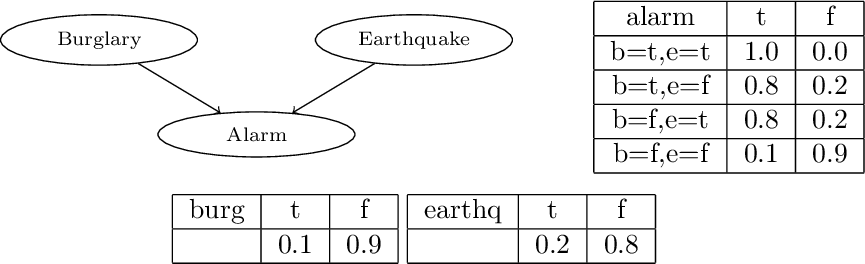burg(t):0.1 ; burg(f):0.9.
earthq(t):0.2 ; earthq(f):0.8.
alarm(t):-burg(t),earthq(t).
alarm(t):0.8 ; alarm(f):0.2:-burg(t),earthq(f).
alarm(t):0.8 ; alarm(f):0.2:-burg(f),earthq(t).
alarm(t):0.1 ; alarm(f):0.9:-burg(f),earthq(f).

# Monty Hall Puzzle

• A player is given the opportunity to select one of three closed doors, behind one of which there is a prize.

• Behind the other two doors are empty rooms.

• Once the player has made a selection, Monty is obligated to open one of the remaining closed doors which does not contain the prize, showing that the room behind it is empty.

• He then asks the player if he would like to switch his selection to the other unopened door, or stay with his original choice.

• Does it matter if he switches?

# Monty Hall Puzzle

:- use_module(library(pita)).
:- endif.
:- pita.
prize(1):1/3; prize(2):1/3; prize(3):1/3.
selected(1).
open_door(A):0.5; open_door(B):0.5:-
member(A,[1,2,3]), member(B,[1,2,3]),
A<B, \+ prize(A), \+ prize(B),
\+ selected(A), \+ selected(B).
open_door(A):-
member(A,[1,2,3]), \+ prize(A),
\+ selected(A), member(B,[1,2,3]),
prize(B), \+ selected(B).
win_keep:-
selected(A), prize(A).
win_switch:-
member(A,[1,2,3]),
\+ selected(A), prize(A),
\+ open_door(A).
:- end_lpad.

# Monty Hall Puzzle

• Queries:

prob(win_keep,Prob).
prob(win_switch,Prob).

# Bongard Problems

• Introduced by the Russian scientist M. Bongard

• Pictures, some positive and some negative

• Problem: discriminate between the two classes.

• The pictures contain shapes with different properties, such as small, large, pointing down, … and different relationships between them, such as inside, above, …# Bongard Problems in cplint

:-use_module(library(slipcover)).
:- if(current_predicate(use_rendering/1)).
:- use_rendering(c3).
:- endif.
:-sc.
:- set_sc(megaex_bottom,20).
:- set_sc(max_iter,3).
:- set_sc(max_iter_structure,10).
:- set_sc(maxdepth_var,4).
:- set_sc(verbosity,1).
bg([]).
in([
(pos:0.5 :-
circle(A),
in(B,A)),
(pos:0.5 :-
circle(A),
triangle(B))]).

# Bongard Problems in cplint

fold(train,[2,3,5,...]).
fold(test,[490,491,494,...]).
output(pos/0).
input_cw(triangle/1).
input_cw(square/1).
input_cw(circle/1).
input_cw(in/2).
input_cw(config/2).
determination(pos/0,triangle/1).
determination(pos/0,square/1).
determination(pos/0,circle/1).
determination(pos/0,in/2).
determination(pos/0,config/2).
modeh(*,pos).
modeb(*,triangle(-obj)).
modeb(*,square(-obj)).
modeb(*,circle(-obj)).
modeb(*,in(+obj,-obj)).
modeb(*,in(-obj,+obj)).
modeb(*,config(+obj,-#dir)).

# Bongard Problems (Models Encoding)

begin(model(2)).
pos.
triangle(o5).
config(o5,up).
square(o4).
in(o4,o5).
circle(o3).
triangle(o2).
config(o2,up).
in(o2,o3).
triangle(o1).
config(o1,up).
end(model(2)).

begin(model(3)).
neg(pos).
circle(o4).
circle(o3).
in(o3,o4).
....

# Bongard Problems (Keys Encoding)

pos(2).
triangle(2,o5).
config(2,o5,up).
square(2,o4).
in(2,o4,o5).
circle(2,o3).
triangle(2,o2).
config(2,o2,up).
in(2,o2,o3).
triangle(2,o1).
config(2,o1,up).

neg(pos(3)).
circle(3,o4).
circle(3,o3).
in(3,o3,o4).
square(3,o2).
circle(3,o1).
in(3,o1,o2).
....

# Bongard Problems

• Parameter learning

induce_par([train],P),
test(P,[test],LL,AUCROC,ROC,AUCPR,PR).
• Structure learning

induce([train],P),
test(P,[test],LL,AUCROC,ROC,AUCPR,PR).

# Mutagenesis in cplint

:- use_module(library(slipcover)).
:- if(current_predicate(use_rendering/1)).
:- use_rendering(c3).
:- endif.
:-sc.
:-set_sc(megaex_bottom,4).
:-set_sc(neg_ex,given).
bg([]).
in([
(active:0.5 :-
lumo(A),
bond(B,C,2),
atm(C,n,32,D)),
(active:0.5 :-
lumo(A),
atm(B,o,40,C),
atm(D,n,32,C)),
...]).
fold(1,[d18,...]).
...
fold(10,[d48,...]).

# Mutagenesis

output(active/0).
input_cw(lumo/1).
input_cw(logp/2).
input_cw(bond/3).
input_cw(atm/4).
input_cw(benzene/1).
input_cw(carbon_5_aromatic_ring/1).
input_cw(carbon_6_ring/1).
input_cw(hetero_aromatic_6_ring/1).
input_cw(hetero_aromatic_5_ring/1).
input_cw(ring_size_6/1).
input_cw(ring_size_5/1).
input_cw(nitro/1).
input_cw(methyl/1).
input_cw(anthracene/1).
input_cw(phenanthrene/1).
input_cw(ball3/1).

# Mutagenesis

modeh(1,active).

modeb(1,lumo(-energy)).
modeb(1,logp(-hydrophob)).
modeb(*,atm(-atomid,-#element,-#int,-charge)).
modeb(*,bond(-atomid,-atomid,-#int)).
modeb(1,(+charge) >= (#charge)).
modeb(1,(+charge) =< (#charge)).
modeb(1,(+charge)= #charge).
modeb(1,(+hydrophob) >= (#hydrophob)).
modeb(1,(+hydrophob) =< (#hydrophob)).
modeb(1,(+hydrophob)= #hydrophob).
modeb(1,(+energy) >= (#energy)).
modeb(1,(+energy) =< (#energy)).
modeb(1,(+energy)= #energy).

# Mutagenesis

modeb(*,benzene(-ring)).
modeb(*,carbon_5_aromatic_ring(-ring)).
modeb(*,carbon_6_ring(-ring)).
modeb(*,hetero_aromatic_6_ring(-ring)).
modeb(*,hetero_aromatic_5_ring(-ring)).
modeb(*,ring_size_6(-ring)).
modeb(*,ring_size_5(-ring)).
modeb(*,nitro(-ring)).
modeb(*,methyl(-ring)).
modeb(*,anthracene(-ringlist)).
modeb(*,phenanthrene(-ringlist)).
modeb(*,ball3(-ringlist)).
modeb(*,member(-ring,+ringlist)).
modeb(1,member(+ring,+ringlist)).

# Mutagenesis

lookahead(logp(B),[(B=_C)]).

determination(active/0,lumo/1).
determination(active/0,logp/2).
determination(active/0,bond/3).
determination(active/0,atm/4).
determination(active/0,benzene/1).
determination(active/0,carbon_5_aromatic_ring/1).
determination(active/0,carbon_6_ring/1).
determination(active/0,hetero_aromatic_6_ring/1).
determination(active/0,hetero_aromatic_5_ring/1).
determination(active/0,ring_size_6/1).
determination(active/0,ring_size_5/1).
determination(active/0,nitro/1).
determination(active/0,methyl/1).
...

# Mutagenesis (Keys)

% fold 1
active(d18).
active(d26).
active(d28).
....

neg(active(d38)).
neg(active(d84)).
neg(active(d100)).
...

# Conclusions

• Exciting field!

• Much is left to do:

• Lifted inference

• Continuous variables

• Structure learning search strategies# References

• Dantsin, E. (1991). Probabilistic logic programs and their semantics. In Russian Conference on Logic Programming, volume 592 of LNCS, pages 152-164. Springer.

• De Raedt, L., Kimmig, A., and Toivonen, H. (2007). Problog: A probabilistic prolog and its application in link discovery. In International Joint Conference on Artificial Intelligence, pages 2462-2467.

• Fierens, D., den Broeck, G.V., Renkens, J., Shterionov, D.S., Gutmann, B., Thon, I., Janssens, G., De Raedt, L.: Inference and learning in probabilistic logic pro- grams using weighted boolean formulas. Theory and Practice of Logic Program- ming 15(3), 358-401 (2015)

# References

• Gutmann, B., Thon, I., and Raedt, L. D. (2011). Learning the parameters of probabilistic logic programs from interpretations. In European Conference on Machine Learning and Knowledge Discovery in Databases, volume 6911 of LNCS, pages 581-596. Springer.

• Ishihata, M., Kameya, Y., Sato, T., and Minato, S. (2008). Propositionalizing the em algorithm by bdds. In Late Breaking Papers of the 18th International Conf. on Inductive Logic Programming, pages 44-49.

• Meert, W., Struyf, J., and Blockeel, H. (2009). CP-Logic theory inference with contextual variable elimination and comparison to bdd based inference methods. In ILP 2009.

# References

• Meert, W., Taghipour, N., and Blockeel, H. (2010). First-order bayes-ball. In Balcázar, J. L., Bonchi, F., Gionis, A., and Sebag, M., editors, Machine Learning and Knowledge Discovery in Databases, European Conference, ECML PKDD 2010, Barcelona, Spain, September 20-24, 2010, Proceedings, Part II, volume 6322 of Lecture Notes in Computer Science, pages 369-384. Springer.

• Muggleton, S. (1995). Inverse entailment and progol. New Generation Comput., 13(3&4):245-286.

• Poole, D. (1993). Logic programming, abduction and probability - a top-down anytime algorithm for estimating prior and posterior probabilities. New Gener. Comput., 11(3):377-400.

• Poole, D. (1997). The Independent Choice Logic for modelling multiple agents under uncertainty. Artif. Intell., 94(1-2):7-56.

# References

• Riguzzi, F. (2007). A top down interpreter for LPAD and CP-logic. In Congress of the Italian Association for Artificial Intelligence, number 4733 in LNAI, pages 109-120. Springer.

• Riguzzi, F. (2009). Extended semantics and inference for the Independent Choice Logic. Logic Journal of the IGPL.

• Riguzzi, F. and Swift, T. (2010). Tabling and Answer Subsumption for Reasoning on Logic Programs with Annotated Disjunctions. In Hermenegildo, M. and Schaub, T., editors, Technical Communications of the 26th Int’l. Conference on Logic Programming (ICLP10), volume 7 of Leibniz International Proceedings in Informatics (LIPIcs), pages 162-171, Dagstuhl, Germany. Schloss Dagstuhl-Leibniz-Zentrum fuer Informatik.

# References

• Sato, T. (1995). A statistical learning method for logic programs with distribution semantics. In International Conference on Logic Programming, pages 715-729.

• Shachter, R. D. (1998). Bayes-ball: The rational pastime (for determining irrelevance and requisite information in belief networks and influence diagrams. In In Uncertainty in Artificial Intelligence, pages 480-487. Morgan Kaufmann.

• Thon, I., Landwehr, N., and Raedt, L. D. (2008). A simple model for sequences of relational state descriptions. In Daelemans, W., Goethals, B., and Morik, K., editors, Machine Learning and Knowledge Discovery in Databases, European Conference, ECML/PKDD 2008, Antwerp, Belgium, September 15-19, 2008, Proceedings, Part II, volume 5212 of Lecture Notes in Computer Science, pages 506-521. Springer.

# References

• Vennekens, J., Verbaeten, S., and Bruynooghe, M. (2004). Logic programs with annotated disjunctions. In International Conference on Logic Programming, volume 3131 of LNCS, pages 195-209. Springer.